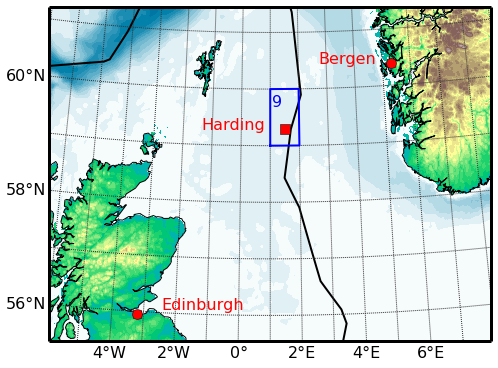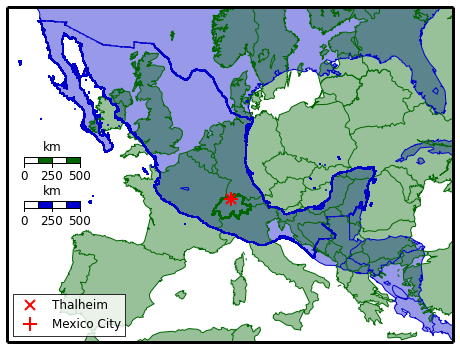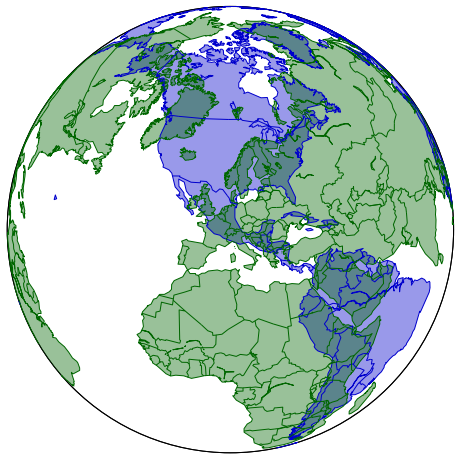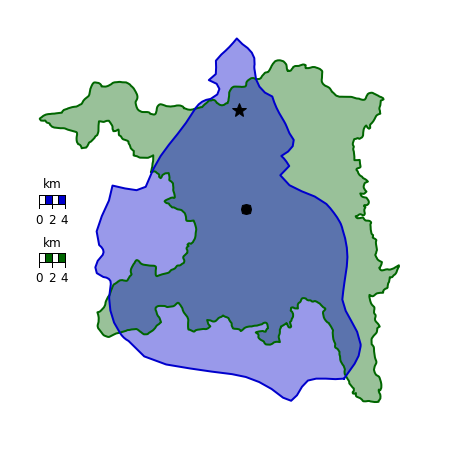# A basemap example

#### 10 September 2014

In this first entry of the aftershock series I show a basemap-example. Not that there would not be enough basemap-examples out in the wild; a quick search on the internet reveals many beautiful ones. But you know how it is: there is always something that you want to do differently than the examples that you find. So you go and look at many different ones, start to mix and match, and end up with your own version that you are happy with. I post my final version here for this very reason, it might be one piece in your own jigsaw. (I deliberately call it an example, not a tutorial, as I do not provide enough information nor do I break it down neatly into simple steps to deserve the tag tutorial.)

But before I can go on to the example we have to install the basemap package. After I had already some troubles installing numpy, scipy, matplotlib, and ipython in a virtual environment, I came across more difficulties when I tried to install basemap in a virtual environment. (If you want to install it system-wide it should not be a problem, just use apt-get install python-mpltoolkits.basemap, or your system’s equivalent for apt-get. However, in a virtual environment it is a bit more complicated.)

During my Ph.D. I used the Enthought Python Distribution (EPD) (now called Enthought Canopy) with an academic license, and I was fairly happy with it. However, after I finished I had no need for a distribution, and just used apt-get for the few packages I needed. But recently I started to have dedicated virtual environments for specific tasks. And there it can be painful at times to set up everything; not the least numpy, scipy, matplotlib, ipython, and, what I need here, basemap. It was just now that I came across Anaconda, a completely free Python distribution, that installs into its own virtual environment. And it is dead easy! I won’t delve into a discussion Canopy vs. Anaconda vs. pick your distribution. I just got to know Anaconda, gave it a shot, and so far I am very pleased with it.

To install Anaconda, download the bash script from Continuum Analytics, and then run it, in my case

$bash Anaconda3-2.0.1-Linux-x86_64.sh  and follow the instructions (the default install-directory is ~/anaconda3; I changed that to ipynb during installation, to be consistent with my previous article). You can then activate and deactivate the environment with $ source ~/ipynb/bin/activate ~/ipynb
$source deactivate  and you are good to go with numpy and friends! The download takes a few minutes, but that is the biggest hurdle in the whole process. What we are still missing for this example is the basemap toolkit. With Anaconda this is, again, very easy to install: (ipynb)$ conda install basemap


For some shapefiles the basemap-function readshapefile had encoding issues that I could not figure out. I used therefore another module, shapefile, that had no such issues. This module is not in the conda-repo, but you can install it with pip in your anaconda environment:

(ipynb) pip install pyshp


Now back to the purpose of this article: a basemap example.

## Plotting the location of the North Sea Harding Oil and Gas field

This example is taken out of my Ph.D. thesis. It shows the North Sea bathymetry and the topography of the surrounding countries, the locations of Edinburgh, Bergen, and the Harding Oil and Gas field, the outline of Block 9 (the hydrocarbon exploration areas are divided into blocks, which are made up of the 1° longitudes and latitudes). It also includes the median line, which defines the economic sectors of the countries adjacent to the North Sea.

All data I use here are freely available online:
• etopo1_bedrock.asc: You can download the etopo-data from the U.S. National Geophysical Data Center (NGDC). For this example, I downloaded a data set with the following parameters:
1. ETOPO1 (bedrock)
2. -8 West to 10 East, 64 North to 54 South
3. ArcGIS ASCII Grid
• DECC_OFF_Median_Line: The median line is downloaded from the UK Department of Energy & Climate Change (DECC). The coordinates of Harding are from Well 9/23b-7, which are also available at the DECC.
• The coordinates of Edinburgh and Bergen are taken from Wikipedia.
In :
import shapefile
import numpy as np
from matplotlib import cm, rcParams
import matplotlib.pyplot as plt
from mpl_toolkits.basemap import Basemap
rcParams.update({'font.size': 16}) # Increase font-size


In :
# Load the topo file to get header information
etopo1name = 'data/basemap/etopo1_bedrock.asc'
topo_file = open(etopo1name, 'r')

# Read header (number of columns and rows, cell-size, and lower left coordinates)
topo_file.close()

# Read in topography as a whole, disregarding first five rows (header)

# Data resolution is quite high. I decrease the data resolution
# to decrease the size of the final figure
dres = 2

# Swap the rows
etopo[:nrows+1, :] = etopo[nrows+1::-1, :]
etopo = etopo[::dres, ::dres]

# Create longitude and latitude vectors for etopo
lons = np.arange(xllcorner, xllcorner+cellsize*ncols, cellsize)[::dres]
lats = np.arange(yllcorner, yllcorner+cellsize*nrows, cellsize)[::dres]


### Create the figure

In :
fig = plt.figure(figsize=(8, 6))

# Create basemap, 870 km east-west, 659 km north-south,
# intermediate resolution, Transverse Mercator projection,
# centred around lon/lat 1°/58.5°
m = Basemap(width=870000, height=659000,
resolution='i', projection='tmerc',
lon_0=1, lat_0=58.5)

# Draw coast line
m.drawcoastlines(color='k')

# Draw continents and lakes
m.fillcontinents(lake_color='b', color='none')

# Draw a think border around the whole map
m.drawmapboundary(linewidth=3)

# Convert etopo1 coordinates lon/lat in ° to x/y in m
# (From the basemap help: Calling a Basemap class instance with the arguments
# lon, lat will convert lon/lat (in degrees) to x/y map projection coordinates
# (in meters).)
rlons, rlats = m(*np.meshgrid(lons,lats))

# Draw etopo1, first for land and then for the ocean, with different colormaps
llevels = np.arange(-500,2251,100) # check etopo.ravel().max()
lcs = m.contourf(rlons, rlats, etopo, llevels, cmap=cm.terrain)
olevels = np.arange(-3500,1,100) # check etopo.ravel().min()
cso = m.contourf(rlons, rlats, etopo, olevels, cmap=cm.ocean)

# Draw parallels and meridians
m.drawparallels(np.arange(-56,63.,2.), color='.2', labels=[1,0,0,0])
m.drawparallels(np.arange(-55,63.,2.), color='.2', labels=[0,0,0,0])
m.drawmeridians(np.arange(-6.,12.,2.), color='.2', labels=[0,0,0,1])
m.drawmeridians(np.arange(-7.,12.,2.), color='.2', labels=[0,0,0,0])

# Draw Block 9 boundaries
m.plot([1, 2, 2, 1, 1], [59, 59, 60, 60, 59], 'b', linewidth=2, latlon=True)
plt.annotate('9', m(1.1, 59.7), color='b')

# Draw maritime boundaries

# 1. Convert coordinates
EDIx, EDIy = m(-3.188889, 55.953056)
BERx, BERy = m(5.33, 60.389444)
HARx, HARy = m(1.5, 59.29)
# 2. Plot symbol
plt.plot(HARx, HARy, mfc='r', mec='k', marker='s', markersize=10)
plt.plot(EDIx, EDIy, mfc='r', mec='k', marker='o', markersize=10)
plt.plot(BERx, BERy, mfc='r', mec='k', marker='o', markersize=10)
# 3. Plot name
plt.text(EDIx+50000, EDIy+10000,'Edinburgh', color='r')
plt.text(BERx-140000, BERy, 'Bergen', color='r')
plt.text(HARx-160000, HARy, 'Harding', color='r')

plt.show()The original intent was to just post the above example a few days ago. However, playing around with basemap got me distracted (it always happens with basemap, there are so many interesting things to do). My imminent move from Scotland to Mexico was a good excuse to play around a bit more with maps.

## Plotting the outline of Mexico and Switzerland on top of each other

I wanted to plot Mexico and Switzerland on top of each other, with the same scale, to compare the size and relative distances. (If you try that in Google maps you run into troubles, as the scale is not the same; try it!)

There is nowadays a wealth of data in the Open Domain when it comes to administrative borders. For this example I use the following data:
• General country boundaries: In-built from the basemap module.
• MEX_adm0, CHE_adm0, CHE_adm1: Borders of Mexico, Switzerland, and the Swiss district Aargau are taken from Global Administrative Areas [GADM]. I think the data from Switzerland are the detailed data from Swisstopo.
• ne_10m_admin_1_states_provinces: I took the border of Mexico City from Natural Earth (the one from GADM was not as detailed as this one).

More resources can be found on the wiki of the Open Street Map, the U.S. Humanitarian Information Unit [HIU], and an internet search will provide many more.

In :
import shapefile
import numpy as np
import matplotlib.pyplot as plt
from mpl_toolkits.basemap import Basemap


Settings for both figures

I want that Mexico City and my home village Thalheim overlay (I took the coordinates from Wikipedia). The easiest way to achieve this is to use them as the centre of the map. However, I shift them subsequently by 2° to the south, as Mexico City is not in the centre of Mexico.

In :
# Coordinates (from Wikipedia) and shift
DF = [-99.1333, 19.4333]
TH = [  8.1042, 47.4375]
shift = [0, 2]

# Colours
cMX = '#0000cc' # Blue tone
cCH = '#006600' # Green tone


Figure 1.A: Transverse Mercator

In :
# 4000 km east-west, 3000 km north-south, low resolution, Transverse Mercator
width = 4000000
height = 3000000
res = 'l'
proj = 'tmerc'

fig1a = plt.figure(figsize=(8, 6))

# Create basemaps for Mexico and Switzerland
m_mx = Basemap(width=width, height=height, resolution=res, projection=proj,
lon_0=DF+shift, lat_0=DF+shift)
m_ch = Basemap(width=width, height=height, resolution=res, projection=proj,
lon_0=TH+shift, lat_0=TH+shift)

# Draw coast lines
m_mx.drawcoastlines(color=cMX, linewidth=1)
m_ch.drawcoastlines(color=cCH, linewidth=1)

# Draw all country borders
m_mx.drawcountries(color=cMX, linewidth=1)
m_ch.drawcountries(color=cCH, linewidth=1)

# Fill continents and lakes
m_mx.fillcontinents(lake_color='none', color=cMX, alpha=.4)
m_ch.fillcontinents(lake_color='none', color=cCH, alpha=.4)

# Draw extra points for the Swiss and Mexican countries to highlight

m_ch.plot(chlonlat[:, 0], chlonlat[:, 1] , '.', c=cCH, latlon=True, ms=1)
m_mx.plot(mxlonlat[:, 0], mxlonlat[:, 1], '.', c=cMX, latlon=True, ms=1)

# Draw scales to cross-check that the scales are the same
# (shift scale 1600 km to the west from centre, and a bit north/south)
smx = np.array(m_mx(DF, DF)) - np.array([1600000,  50000])
sch = np.array(m_ch(TH, TH)) - np.array([1600000, -350000])
ismx = m_mx(smx, smx, inverse='True')
isch = m_ch(sch, sch, inverse='True')

m_mx.drawmapscale(ismx, ismx, DF+shift, DF+shift,
500, barstyle='fancy', fillcolor2=cMX, fontsize=12)
m_ch.drawmapscale(isch, isch, TH+shift, TH+shift,
500, barstyle='fancy',  fillcolor2=cCH, fontsize=12)

# Add Mexico City and Thalheim AG
m_mx.plot(DF, DF, 'x', c='r', ms=10, mew=2, latlon=True, label='Thalheim')
m_ch.plot(TH, TH, '+', c='r', ms=14, mew=2, latlon=True, label='Mexico City')
lg = plt.legend(loc='lower left', fontsize=12, numpoints=1)
lg.get_frame().set_alpha(.8) # A little transparency

# Draw a thick border around the whole
m_mx.drawmapboundary(color='k', linewidth=3)

plt.show()Figure 1.B: Orthographic

In :
fig1b = plt.figure(figsize=(8, 8))

# Create basemaps for Mexico and Switzerland
m_mx = Basemap(resolution='c', projection='ortho', lon_0=DF, lat_0=DF)
m_ch = Basemap(resolution='c', projection='ortho', lon_0=TH, lat_0=TH)

# Draw coast lines
m_mx.drawcoastlines(color=cMX, linewidth=1)
m_ch.drawcoastlines(color=cCH, linewidth=1)

# Draw all country borders
m_mx.drawcountries(color=cMX, linewidth=1)
m_ch.drawcountries(color=cCH, linewidth=1)

# Fill continents and lakes
m_mx.fillcontinents(lake_color='none', color=cMX, alpha=.4)
m_ch.fillcontinents(lake_color='none', color=cCH, alpha=.4)

plt.show()### B. Districts

Figure 2

In :
# 70 km by 70 km, Transverse Mercator, resolution does not
# matter, as we use other data for the borders
width = 70000
height = 70000
dshift = [-.133, -.05]

fig2 = plt.figure(figsize=(8,8))

# Create basemaps for Mexico and Switzerland
m_mx = Basemap(width=width, height=height, projection=proj,
lon_0=DF, lat_0=DF+dshift)
m_ch = Basemap(width=width, height=height, projection=proj,
lon_0=TH, lat_0=TH+dshift)

# Draw the district of Aargau
iag = [i for i, s in enumerate(CHE_adm1.records()) if 'Aargau' in s]
m_ch.plot(aglonlat[:, 0], aglonlat[:, 1], '-', c=cCH, latlon=True, lw=2)
agx, agy = m_ch(aglonlat[:, 0], aglonlat[:, 1])
plt.fill(agx, agy, cCH, ec='none', alpha=.4)

# Draw Mexico City DF
idf = [i for i, s in enumerate(MEX_adm1.records()) if 'MX.DF' in s]
m_mx.plot(dflonlat[:, 0], dflonlat[:, 1], '-', c=cMX, latlon=True, lw=2)
dfx, dfy = m_mx(dflonlat[:, 0], dflonlat[:, 1])
plt.fill(dfx, dfy, cMX, ec='none', alpha=.4)

# Draw scales to cross-check that the scales are the same
sdf = np.array(m_mx(DF, DF)) - np.array([28000, 10000])
sth = np.array(m_ch(TH, TH)) - np.array([28000, 10000])
isdf = m_mx(sdf, sdf, inverse='True')
isth = m_ch(sth, sth, inverse='True')
m_mx.drawmapscale(isdf, isdf, DF, DF+dshift,
4, barstyle='fancy', fillcolor2=cMX, fontsize=12)
m_ch.drawmapscale(isth, isth, TH, TH+dshift,
4, barstyle='fancy',  fillcolor2=cCH, fontsize=12)

# Draw locations
hPT = [-99.12, 19.47]
pPT = [-99.11, 19.33]
m_mx.plot(hPT, hPT, '*', c='k', ms=14, mew=1, latlon=True)
m_mx.plot(pPT, pPT, 'o', c='k', ms=10, mew=1, latlon=True)

# Remove border around the whole
m_mx.drawmapboundary(color='none')

plt.show()Mexico City (blue) and my home district Aargau (green) are approximately the same size (Wikipedia: DF ~1485 km2, AG ~1404 km2). True, roughly a third to the south and the south-west of the above outline of Mexico City is mountainous area. But then the city extends to the east and the north outside the official DF-boundaries, so the area-comparison roughly holds.

In Mexico, when we go from home (*) to my parents in law (o), we make about the same distance as if we would go from Thalheim to the German border, just without ever leaving the city. Interesting indeed.

As usual, the notebooks with the above code, Basemap.ipynb and MXCH.ipynb, can be found on my GitHub page in the blog-notebooks-repo.

Update: You can find more basemap examples in the Travel Map post.